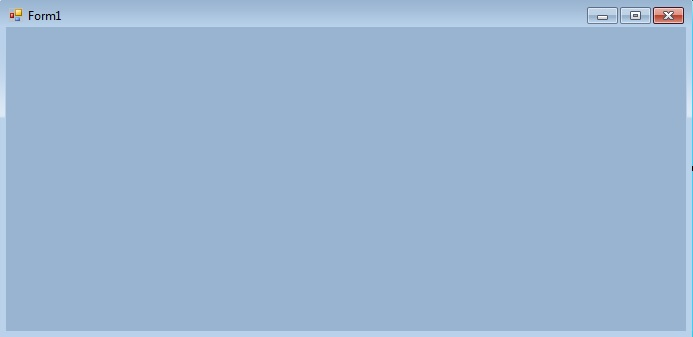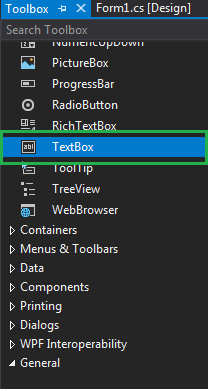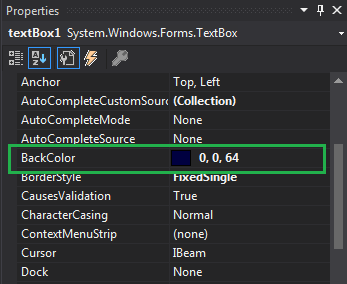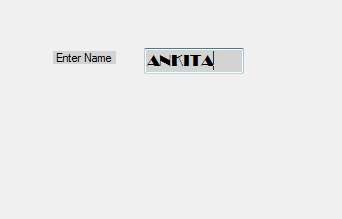Related Articles
How to set the background color of the TextBox in C#?
• Last Updated : 29 Nov, 2019

In Windows forms, TextBox plays an important role. With the help of TextBox, the user can enter data in the application, it can be of a single line or of multiple lines. In TextBox, you are allowed to set the background color of the TextBox with the help of BackColor property which makes your textbox more attractive. In Windows form, you can set this property in two different ways:

1. Design-Time: It is the simplest way to set the BackColor property of the TextBox. As shown in the following steps:

• Step 1: Create a windows form. As shown in the below image:
Visual Studio -> File -> New -> Project -> WindowsFormApp• Step 2: Drag the TextBox control from the ToolBox and Drop it on the windows form. You can place TextBox anywhere on the windows form according to your need. As shown in the below image:• Step 3: After drag and drop you will go to the properties of the TextBox control to set the BackColor property of the TextBox. As shown in the below image:Output:2. Run-Time: It is a little bit trickier than the above method. In this method, you can set the BackColor property of the TextBox programmatically with the help of given syntax:

`public override System.Drawing.Color BackColor { get; set; }`

Here, Color is used to represent the background color of the TextBox. Following steps are used to set the BackColor property of the TextBox:

• Step 1 : Create a textbox using the TextBox() constructor provided by the TextBox class.
```// Creating textbox
TextBox Mytextbox = new TextBox();
```
• Step 2 : After creating TextBox, set the BackColor property of the TextBox provided by the TextBox class.
```// Set BackColor property
Mytextbox.BackColor = Color.LightGray;
```
• Step 3 : And last add this textbox control to from using Add() method.
```// Add this textbox to form
 `using` `System;``using` `System.Collections.Generic;``using` `System.ComponentModel;``using` `System.Data;``using` `System.Drawing;``using` `System.Linq;``using` `System.Text;``using` `System.Threading.Tasks;``using` `System.Windows.Forms;`` ` `namespace` `my {`` ` `public` `partial` `class` `Form1 : Form {`` ` `    ``public` `Form1()``    ``{``        ``InitializeComponent();``    ``}`` ` `    ``private` `void` `Form1_Load(``object` `sender, EventArgs e)``    ``{``        ``// Creating and setting the properties of Lable1``        ``Label Mylablel = ``new` `Label();``        ``Mylablel.Location = ``new` `Point(96, 54);``        ``Mylablel.Text = ``"Enter Name"``;``        ``Mylablel.AutoSize = ``true``;``        ``Mylablel.BackColor = Color.LightGray;`` ` `        ``// Add this label to form``        ``this``.Controls.Add(Mylablel);`` ` `        ``// Creating and setting the properties of TextBox1``        ``TextBox Mytextbox = ``new` `TextBox();``        ``Mytextbox.Location = ``new` `Point(187, 51);``        ``Mytextbox.BackColor = Color.LightGray;``        ``Mytextbox.AutoSize = ``true``;``        ``Mytextbox.Name = ``"text_box1"``;``        ``Mytextbox.Font = ``new` `Font(``"Broadway"``, 12);`` ` `        ``// Add this textbox to form``        ``this``.Controls.Add(Mytextbox);``    ``}``}``}`